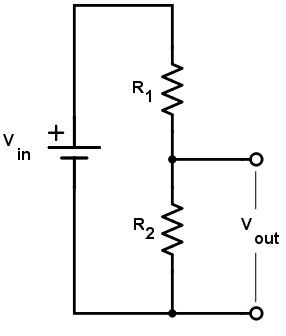# Voltage Divider Calculator

222

### Use this tool to calculate the output voltage of a resistor divider circuit for a given set of resistor values and source voltage

V

V×